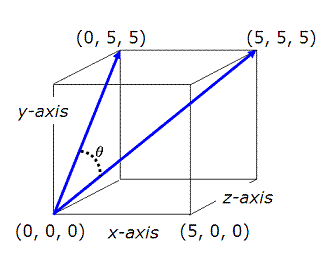# Angle between vectors

This article describes how to calculate the angle between vectors, the angle between each vector and axis, and the magnitude of each vector. The vectors are given in three-dimensional space. The code and examples were developed in Matlab code.

 We are going to enter the tip of the vectors assuming that they both start at the origin point (0, 0, 0). For example, to enter vector V1 that goes from (0, 0, 0) to (3, 4, 7) we have to enter just the last point, in a row array in Matlab, like this: v1 = [3 4 7] We now develop a function to calculate the values, and another function to display those values, in order to be

able to call those scripts whenever we need them and avoid retyping instructions for every couple of vectors that we use.

The script to find relevant values is:

function [m, a] = ab2v(v1, v2)
% Magnitude of vector is its norm
m(1) = norm(v1);
m(2) = norm(v2);

% Min. angles from vector 1 to axes
a(1 : 3) = acosd(v1 / m(1));
% Min. angles from vector 2 to axes
a(4 : 6) = acosd(v2 / m(2));

% Dot product dot(v1, v2) equals sum(v1 .* v2)
a(7) = acosd(dot(v1, v2) / m(1) / m(2));

The m-file to display and format those values is:

function display_angles(m, a)

disp(' ')
disp([
'Angle between vectors: ' num2str(a(7))])

disp(' ')
disp(
'Vector 1')
disp([
'Magnitude :' num2str(m(1))])
disp([
'Min. angle with x-axis: ' num2str(a(1))])
disp([
'Min. angle with y-axis: ' num2str(a(2))])
disp([
'Min. angle with z-axis: ' num2str(a(3))])

disp(' ')
disp(
'Vector 2')
disp([
'Magnitude :' num2str(m(2))])
disp([
'Min. angle with x-axis: ' num2str(a(4))])
disp([
'Min. angle with y-axis: ' num2str(a(5))])
disp([
'Min. angle with z-axis: ' num2str(a(6))])

Built-in function ‘num2str’ converts numbers to strings, in order to concatenate them to other strings and improve the formatting previously to display the results on screen.

Example 1:

Find the angle between a diagonal of a cube and a diagonal of one of its faces. The cube measures 5x5x5 units.We finally create a third Matlab script, to try our functions above and to drive them effortlessly. This gives us an easy way to handle all the examples that we’d like to test...

clc; clear; close all; format compact

v1 = [0 5 5]
v2 = [5 5 5]

[m, a] = ab2v(v1, v2);
display_angles(m, a)

The on-screen result is:

Angle between vectors: 35.2644

Vector 1
Magnitude :7.0711
Min. angle with x-axis: 90
Min. angle with y-axis: 45
Min. angle with z-axis: 45

Vector 2
Magnitude :8.6603
Min. angle with x-axis: 54.7356
Min. angle with y-axis: 54.7356
Min. angle with z-axis: 54.7356

Example 2:

Find out the angle between vectors (1, 0, 0) and (1, 1, 0).

It’s nice to have this case as an example, because we can figure it out from the beginning, right?

We run our driver:

clc; clear; close all; format compact

v1 = [1 0 0]
v2 = [1 1 0]

[m, a] = ab2v(v1, v2);
display_angles(m, a)

And find the solution:

Angle between vectors: 45

Vector 1
Magnitude :1
Min. angle with x-axis: 0
Min. angle with y-axis: 90
Min. angle with z-axis: 90

Vector 2
Magnitude :1.4142
Min. angle with x-axis: 45
Min. angle with y-axis: 45
Min. angle with z-axis: 90